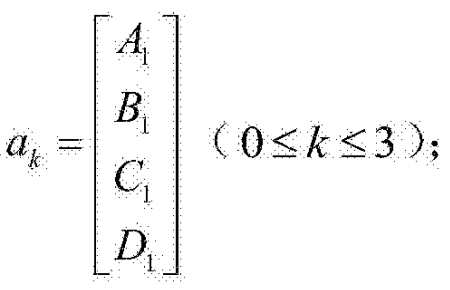QQCustomer Service
Product Catalogue
Tel :
+86-758-2876921
+86-758-2877695
Fax :
+86-758-2838131
QQ :
3004803562/1508496831
Tangxia Industry Zone, Mugang Town, Duanzhou Dist, Zhaoqing City, Guangdong Pro., China
Technical Support
NTC Thermistor Test Method For Automotive Air Conditioning
2019/10/09 03:10:14Thermistor is a kind of resistance with different resistance values according to different temperatures, which is widely used in automotive electronic controllers, especially automotive air conditioning, lithium battery management system (BMS) and automotive power controller, etc., all need to be applied to thermistor module. NTC thermistor refers to the resistance value that decreases with temperature increases, which is widely used in various temperature detection situations because of its temperature sensitivity, high precision and low price. However, the resistance value of NTC thermistor has nonlinear correspondence with temperature, so it’s difficult to be test in practical application.

In the existing automotive air conditioning on the market, there are two main methods to obtain the temperature of the applied NTC thermistor, one is to directly use the ideal B25 value and RT value, using this method, it will make it difficult for the thermistor to set according to the ideal value in actual production, if the actual application of the NTC thermistor is applied electronically according to the ideal value, will cause a large error in the product. The other is to directly input the RT table provided by the thermistor manufacturer into the automotive air conditioning controller, but since the number of RT tables provided by the manufacturer is either very large, it requires software engineers to input one by one, not only a lot of work, but also human error; Either, the values in RT table are few, is directly processed linearly, resulting in a large error. Therefore, it’s necessary to test the thermistor used in automotive air conditioning before it’s put into use to verify the validity of RT table data and ensure that automotive air conditioners have high temperature control accuracy.

Today, let’s introduce an NTC thermistor test method for automotive air conditioning, which selects third-order polynomial fitting formula based on the Steinhart-Hart equation, fitting analysis the data value of RT table provided by the NTC thermistor manufacturer, compare the fitted data with the original data, review the NTC thermistor if the residual errors exceed the pre-set threshold value. In addition, in view of the NTC thermistors with less RT table data, the characteristic curve segment interpolation is adopted to modify the RT table data, and a set of new RT table data suitable for application is given after software filtering, providing a reference for the selection of NTC thermistors. Specific steps are as follows:

(1) Select the third-order polynomial fitting formula for curve fitting of the RT table data for NTC thermistor, as follows:

RT=R25exp (A+B/T+C/T2+D/T3) (1)

OrAmong them, RT is the resistance value of T, T is the temperature of Fahrenheit, R25 is the resistance value at room temperature of 25℃, A, B, C, D, A1, B1, C1 and D1 are constants;

(2) The RT table data value provided by the NTC thermistor manufacturer is converted into a constant matrix (Tincar <i>) j, where i is the number of columns of the matrix, j is the number of rows of the matrix, and (Tincar<0>) j (Can be abbreviated to Tincar<0>) is the temperature parameter in the RT table data;

(3) The constant matrix (Tincar<i>)j is used by software to perform the characteristic analysis;

(4) The error analysis of the temperature parameters of NTC thermistor is carried out by the software to see whether it meets the design requirements of automobile air conditioning;

(5) Convert (Tincar<0>) j into Fahrenheit temperature Tmpm (0≤m≤j-1) (Can be abbreviated to Tmp):

Tmp: =Tincar<0>+273.15 (3)

(6) The data in the constant matrix (Tincar <i>) except Tincar <0>and the calculated Tmpm value are carried into the polynomial fitting formula (2) to calculate the constant A1, B1, C1, D1 and obtain the constant matrix(7) Bringing Tmp and ak into the following formula (4) to calculate the resistance value of the fitted NTC thermistor RT (Tmp·K, ash);(8) The software is used to compare the fitted resistance value RT(Tmp·K, ash) with the RT table data value provided by the NTC thermistor manufacturer and carry out residual analysis. Review the NTC thermistor if the residual errors exceed the pre-set threshold value;

(9)A new set of RT table data suitable for application was obtained through A/D sampling after software filtering, which provided a reference for selecting NTC thermistor. And in a given set of new RT table data suitable for application, the thermistor is given as the emergency temperature data when the wire harness of the temperature sensor breaks.

As an improvement, in the process of curve segment interpolation of RT table data, the equal step data interpolation of the equal step data of step 1 in the temperature value of rt table data is 1, and the resistance value of the remaining temperature range is interpolated with an equal step of 5.

The filtering formula used by the software filtering is:rem[rem+filt*(2^f_rate-1)+input]&(2^f_rate-1)(6)

Among them, filt is the cycle filter value of software filtering, input is the input current value of software filtering, and f-rate is the filter speed of software filtering.

Compared with the traditional method which uses the exponential formula for calculation, this test method is more consistent with the measured data in a larger temperature range. Moreover, the formula used is relatively concise, and the calculation amount of fitting parameters and equation is less, so the calculation speed is faster. This method has higher precision, takes up less storage space of computer and faster computing speed in engineering applications. This test method can also be used to evaluate the NTC thermistor validity of manufacturers’ RT table data and if its precision meets the design requirements, in the thermistor has a certain guiding role of procurement management, to avoid error caused by using ideal B25 value or RT value in product design, the error caused by thermistors to actual products is improved at the same time.

mqu.cn site.nuo.cn